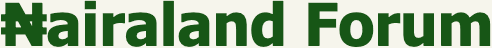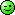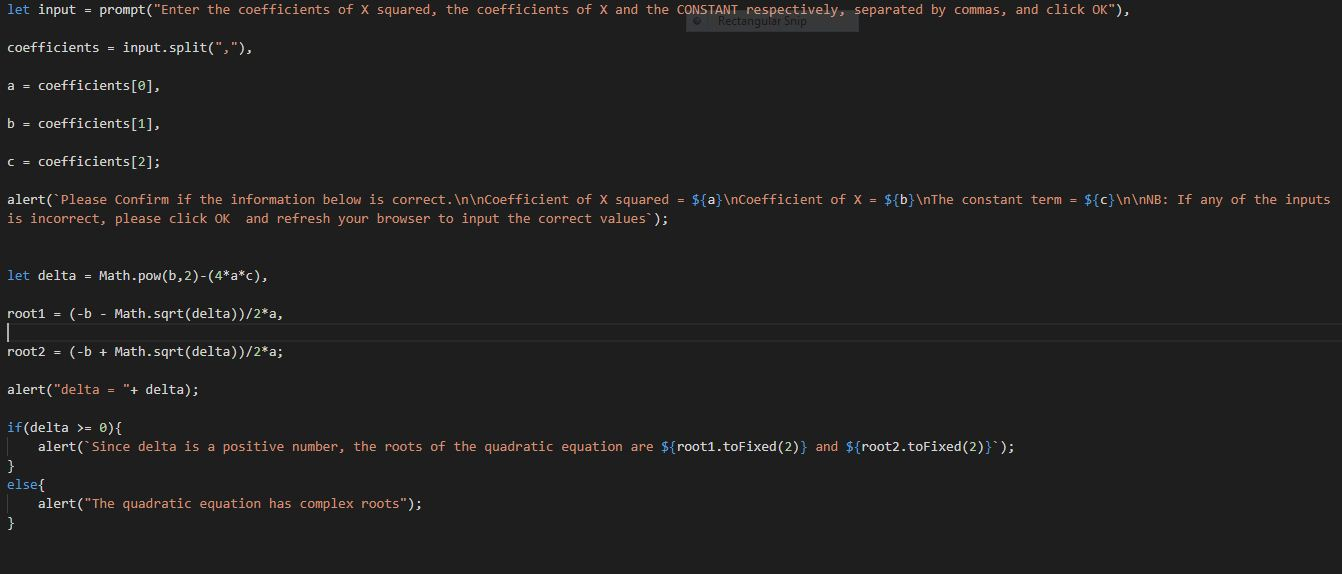#Welcome, Guest: Register On Nairaland / LOGIN! / Trending / Recent / New
Stats: 2,936,995 members, 7,107,336 topics. Date: Friday, 07 October 2022 at 05:43 PM

## Help A Beginner Code This Using Javascript - Programming - Nairaland

(1) (Reply) (Go Down)

 Help A Beginner Code This Using Javascript by Fisayo22: 5:02pm On Jun 06, 2020 write a piece of code using javascript that solves second degree equations. You pass the a, b, c parameters of: ax2 + bx + c, and the solve function will compute delta = b2 - 4a*c. Test the sign of delta, and if it's equal to zero, then display (in the console, or better, in the page) the roots of the equation. 1 Like 1 Share Re: Help A Beginner Code This Using Javascript by Tim1212(m): 6:01pm On Jun 06, 2020 Fisayo22:write a piece of code using javascript that solves second degree equations. You pass the a, b, c parameters of: ax2 + bx + c, and the solve function will compute delta = b2 - 4a*c. Test the sign of delta, and if it's equal to zero, then display (in the console, or better, in the page) the roots of the equation.This is absolutely easy. Drop your email Re: Help A Beginner Code This Using Javascript by Fisayo22: 6:55pm On Jun 06, 2020 idades489@gmail.com Re: Help A Beginner Code This Using Javascript by SUPREMOTM: 8:39pm On Jun 06, 2020 Fisayo22:write a piece of code using javascript that solves second degree equations. You pass the a, b, c parameters of: ax2 + bx + c, and the solve function will compute delta = b2 - 4a*c. Test the sign of delta, and if it's equal to zero, then display (in the console, or better, in the page) the roots of the equation.COPY AND PASTE it in your code editor, then link the javascript file to your HTML file so you can make use of the browser to view the operations. Ask for clarifications wherever you are confused.let input = prompt("Enter the coefficients of X squared, the coefficients of X and the CONSTANT respectively, separated by commas, and click OK",coefficients = input.split(",",a = coefficients,b = coefficients,c = coefficients;alert(`Please Confirm if the information below is correct.\n\nCoefficient of X squared = \${a}\nCoefficient of X = \${b}\nThe constant term = \${c}\n\nNB: If any of the inputs is incorrect, please click OK and refresh your browser to input the correct values`);let delta = Math.pow(b,2)-(4*a*c),root1 = (-b - Math.sqrt(delta))/2*a,root2 = (-b + Math.sqrt(delta))/2*a;alert("delta = "+ delta);if(delta >= 0){ alert(`Since delta is a positive number, the roots of the quadratic equation are \${root1.toFixed(2)} and \${root2.toFixed(2)}`);}else{ alert("The quadratic equation has complex roots";}Re: Help A Beginner Code This Using Javascript by kay9(m): 1:01am On Jun 10, 2020 SUPREMOTM:COPY AND PASTE it in your code editor, then link the javascript file to your HTML file so you can make use of the browser to view the operations. Ask for clarifications wherever you are confused.let input = prompt("Enter the coefficients of X squared, the coefficients of X and the CONSTANT respectively, separated by commas, and click OK",coefficients = input.split(",",a = coefficients,b = coefficients,c = coefficients;alert(`Please Confirm if the information below is correct.\n\nCoefficient of X squared = \${a}\nCoefficient of X = \${b}\nThe constant term = \${c}\n\nNB: If any of the inputs is incorrect, please click OK and refresh your browser to input the correct values`);let delta = Math.pow(b,2)-(4*a*c),root1 = (-b - Math.sqrt(delta))/2*a,root2 = (-b + Math.sqrt(delta))/2*a;alert("delta = "+ delta);if(delta >= 0){ alert(`Since delta is a positive number, the roots of the quadratic equation are \${root1.toFixed(2)} and \${root2.toFixed(2)}`);}else{ alert("The quadratic equation has complex roots";}Nice.I would have given different prompts for each of the 3 coefficients though... As in://Prompt user to input values of a, b, and c...let a = Number(prompt("Please enter the value of a..." ));let b = Number(prompt("Please enter the value of b..." ));let c = Number(prompt("Please enter the value of c..." ));Etc...Simpler and eliminates need for the second alert (i.e. the "Please confirm..." bit).Still, neat coding. Re: Help A Beginner Code This Using Javascript by SUPREMOTM: 2:13am On Jun 10, 2020 kay9:Nice.I would have given different prompts for each of the 3 coefficients though... As in:Simpler and eliminates need for the second alert (i.e. the "Please confirm..." bit).Still, neat coding.Okay Re: Help A Beginner Code This Using Javascript by PrinceDekunle: 9:07am On Jun 10, 2020 Have you considered learning C# which is a top and highly demanded technology of the Microsoft .Net framework?Subscribe to this channel and learn everything for free from the scratch till you become a pro.https://www.youtube.com/channel/UCwNrlP_X_VV4vg00P2QmDnwYou can as well join our Facebook community where we answer questions, discuss and share ideas about technologieshttps://www.facebook.com/groups/738709033333419/?ref=share